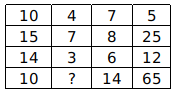Question 17

# Study the given pattern carefully and select the number that can replace the question mark (?) in it.Solution

(15 - 14) $$\times 10 = 1 \times 10$$ = 10
(8 - 6) $$\times 7 = 2 \times 7$$ = 14
(25 - 12) $$\times 5 = 13 \times 5$$ = 65
Similarly,
(7 - 3) $$\times 4 = 4 \times 4$$ = 16
$$\therefore$$ The correct answer is option B.

• Free SSC Study Material - 18000 Questions
• 230+ SSC previous papers with solutions PDF

3 years, 5 months ago

how 16

OR